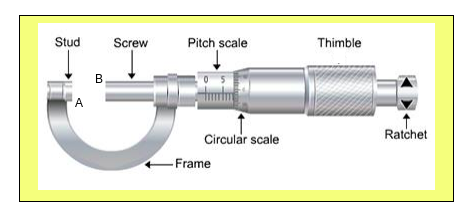#### How to measure diameter of a wire using screw gauge.1.  Note the number of divisions on the circular scale.

2.    Give five complete rotations to the screw.

3.    Note the linear distance moved by the screw.

4.    Find the pitch and L.C. of screw gauge.

5.    Find the zero error and zero correction by moving the screw only in one direction in such a way that studs A and B just touch each other.

6.    Now grip the given wire in the gap A and B of the screw gauge.

7.    Turn the screw head till the ratchet arrangement gives a click.

8.    Note the readings of linear scale and circular scale and find the observed thickness using the relation, observation thickness = L.S.R. + C.S.R.

9.    Add the zero correction to the observed thickness to find the corrected diameter.

10.  Repeat steps 6 to 9 to find the thickness from four more different places.

=L.C = least count